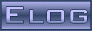Back Midas Rome Roody Rootana
 Midas DAQ System Not logged inMessage ID: 2265     Entry time: 14 Jul 2021     In reply to: 2253     Reply to this: 2266
 Author: Konstantin Olchanski Topic: Bug Fix Subject: changes in history plots
```Moving in the direction of this proposal. History plot editor is updated according to it. Remaining missing piece is the "show
raw value" buttons and code behind them.

Changes:

- "show factor and offset" moved to the top of the page, "off" by default
- factor and offset (if not zero) are automatically migrated to the formula field (if it is empty), one needs to save the panel
for this to take effect.

K.O.

> > I am updating the history plots.
> > So the idea is to use this computation:
> > y_position_on_plot = offset + factor*(formula(history_value) - voffset)
>
> Stefan and myself did some brain storming on zoom. Writing it down the way I remember it.
>
> - we distilled the gist of the problem - the numerical values we show in the plot labels and in hover-over-the-graph
> are before formula is applied or after the formula is applied?
>
> - I suggested a universal solution using a double formula: use formula1 for one case;
>   use formula2 for the other case;
>   use formula1 for "physics calibration", use formula2 for factor and offset for composite plots:
>      numeric_value = formula1(history_value)
>      plotted_value = formula2(numeric_value)
>
> - we agree that this is way too complicated, difficult to explain and difficult to coherently present in the history editor
>
> - Stefan suggested a simple solution, a checkbox labeled "show raw value" next to each history variable. by default, the
> value after the formula is plotted and displayed. if checked, the raw value (before the formula) is displayed, and the
> value after the formula is plotted. (so this works the same as the factor and offset on the old history plots).
>
> - if "show raw value" is enabled, the numerical values shown will be inconsistent against the labels on the vertical axis.
> Our solution it to turn the axis labels off. (for composite plots, like oscillator frequency in Hz vs oscillator
> temperature in degC, both scaled to see their correlation, the vertical axis is unit-less "arbitrary units", of course)
>
> - to simplify migration of old history plots that use custom factor and offset settings, we think in the direction of
> automatically moving them to the "formula". (factor=2, offset=10 automatically populates formula with "2*x+10", "show raw
> value" checked/enabled). Thus we can avoid implementing factor and offset in the new history code (an unwelcome
> complication).
>
> - I think this covers all the use cases I have seen in the past, so we will move in this direction.
>
> K.O.```
ELOG V3.1.4-2e1708b5